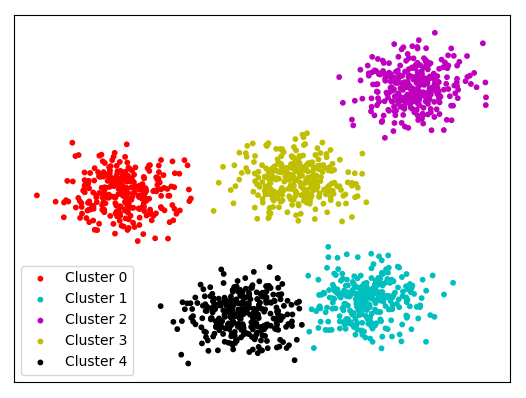# Is Annoy a machine learning algorithm to find nearest neighbor ? and is it similar to K nearest neighbor algorithm?

I was researching about Google universal sentence encoding and i saw that it uses simple neighbor/Annoy to find the nearest vector for semantic-similarity search engine. This is the first time i'm hearing about it. Is simple neighbor/Annoy another kind of machine learning algorithm to find nearest neighbor ? and is it similar to K nearest neighbor algorithm ?

Don't have enough reputation to comment to a resource, so answering this myself.

Annoy is a library being used here for finding approximate nearest neighbours, approximate being the key word here.

## Understanding K-NN and Approximate NN

Now, let's see what is the difference with example of a problem.

Say you have 10 entities (words / sentences / objects / anything) for which you have vectors.

1 vector for each entity.

Let's consider that the metric we're interested in finding for finding neighbours is cosine similarity / cosine distance

How many pairs would this form? Roughly (10 * 9) / 2 = 45.

So, you can find cosine similarity between the 45 pairs and then find K-nearest neighbours you want. Pretty simple. This is your K-Nearest Neighbours algorithm.

Also given you are storing these 45 cosine similarities somewhere, you basically have to deal with storing 45 float numbers (cosine similarities can be between -1 and 1).

Now, let's scale up the problem. What if instead of having 10 entities, you have 10,000,000, i.e. 10M entities. Pretty easy to have that number of entities exist in real world.

Now, you'll need to find cosine similarity between (10M * (10M-1) / 2) = 4,99,99,99,50,00,000 pairs. Which is an enormous number! Basically, the complexity grows with O(n^2), where n is number of entities.

Now, think about finding K-nearest neighbours for any given entity. You'll also be running sorting algorithm for 10M similarities for it. Also, let's just think about storage again. Say 1 float number takes 64 bits (or 8bytes). How much storage do you need to store those many pairs?

4,99,99,99,50,00,000 * 8 bytes ~ 364 TeraBytes!

Getting a memory (RAM) of that size is near to impossible.

So, you understand the problem we're having with regular K-NN approach. We don't wish to calculate all pairs, and it's very difficult to store distances for those many pairs and calculate true K-nearest neighbours at runtime for any entity.

This is where Approximate Nearest Neighbours comes into picture. These say, that given your need of the problem is almost always going to be to get only the K Nearest neighbours, why bother calculating similarities for any given entity to those entities that have zero chances to appear in neighbourhood?Think about a space where you have clusters of points. Say you need to only find the 3 most nearest neighbours for every point for your problem, then you would never need to find similarities between points of different clusters. Right?

This is the idea that Approximate Nearest Neighbour algorithm runs on.

For any given entity, instead of calculating similarities/distances to each of the other point, it chooses X other points and only calculates distances to those.

This reduces the complexity from O(n2) to just O(n*k). Storage becomes easier, Calculation becomes faster.

## A hint of Annoy's implementation

While I'm myself not familiar with all implementation details of Annoy's ANN approach. Usually, most ANN approaches require building a Cluster Index first -- which basically decides the X leaders with which you'll find similarities. Then, the cluster index is used to find Approximate K-nearest neighbours for all given points.

The reason why this is called 'approximate' is that the cluster building process is not perfect. If it were, it'll take days or years of computation and defeat the purpose of reducing complexity. It is approximate cluster indices. Which means, sometimes you can miss out on one of the most similar entity appearing in neighbourhood of given entity. Usually, however this doesn't happen so much that it affects practically. Spotify, Facebook, Google and many large companies are using approximate nearest neighbours approaches.

Annoy is one of the most widely used library for doing approximate nearest neighbours.